# RS Aggarwal Class 6 Solutions Chapter 7 - Decimals Ex 7A (7.1)

## RS Aggarwal Class 6 Chapter 7 - Decimals Ex 7A (7.1) Solutions Free PDF

Convert each of the following into a fraction in its simplest form:

(1) 0.9

We have:

= 0.9 = $\frac {9} {10}$

(2) 0.6

We have:

= 0.6 = $\frac {6} {10} = \frac {3} {5}$

(3) 0.08

We have:

= 0.08 = $\frac {8} {100} = \frac {4} {50} = \frac {2} {25}$

(4) 0.15

We have:

= 0.15 = $\frac {15} {100} = \frac {3} {20}$

(5) 0.48

We have:

= 0.48 = $\frac {48} {100} = \frac {12} {25}$

(6) 0.053

We have:

= 0.053 = $\frac {53} {1000}$

(7) 0.125

We have:

= 0.125 = $\frac {125} {1000} = \frac {25} {200} = \frac {5} {40} = \frac {1} {8}$

(8) 0.224

We have:

= 0.224 = $\frac {224} {1000} = \frac {56} {250} = \frac {28} {125}$

Convert each of the following as a mixed fraction:

(9) 6.4

We have:

= 6.4 = $\frac {64} {10} = \frac {32} {5} = 6 \frac {2} {5}$

(10) 16.5

We have:

= 16.5 = $\frac {165} {10} = \frac {33} {2} = 16 \frac {1} {2}$

(11) 8.36

We have:

= 8.36 = $\frac {836} {100} = \frac {209} {25} = 8 \frac {9} {25}$

(12) 4.275

We have:

= 4.275 = $\frac {4275} {1000} = \frac {171} {40} = 4 \frac {11} {40}$

(13) 25.06

We have:

= 25.06 = $\frac {2506} {100} = \frac {1253} {50} = 25 \frac {3} {50}$

(14) 7.004

We have:

= 7.004 = $\frac {7004} {1000} = \frac {1751} {250} = 7 \frac {1} {250}$

(15) 2.052

We have:

= 2.052 = $\frac{2052}{1000} = \frac{513}{250} = 2\frac{13}{250}$

(16) 3.108

We have:

= 3.108 = $\frac {3108} {1000} = \frac {777} {250} = 3 \frac {27} {250}$

Convert each of the following into decimal:

(17) $\frac {23} {10}$

We have:

$\frac {23} {10}$ = $2 \frac {3} {10}$

= 2 + 0.3 = 2.3

(18) $\frac {167} {100}$

We have:

$\frac {167} {100}$ = $1 \frac {67} {100}$ = 1 + 0.67 = 1.67

(19) $\frac {1589} {100}$

We have:

$\frac {1589} {100}$ = $15 \frac {89} {100}$ = 15 + 0.89 = 15.89

(20) $\frac {5413} {1000}$

We have:

$\frac {5413} {1000}$ = $5 \frac {413} {1000}$ = 5 + 0.413 = 5.413

(21) $\frac {21415} {1000}$

We have:

$\frac {21415} {1000}$ = $21 \frac {415} {1000}$ = 21 + 0.415 = 21.415

(22) $\frac {25} {4}$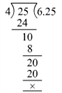We have:

$\frac {25} {4}$ = $6 \frac {1} {4}$ = 6 + 0.25 = 6.25

(23) $3 \frac {3} {5}$

= $\frac {18} {5}$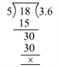We have:

$3 \frac {3} {5}$ = 3 + 0.6 = 3.6

(24) $1 \frac {4} {25}$

= $1 \frac {4} {25}$ = $\frac {29} {25}$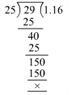We have:

$1 \frac {4} {25}$ = 1 + 0.16 = 1.16

(25) $5 \frac {17} {50}$

= $5 \frac {17} {50}$ = $\frac {267} {50}$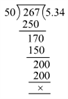We have:

$5 \frac {17} {50}$ = 5 + 0.34 = 5.34

(26) $12 \frac {3} {8}$

$12 \frac {3} {8}$ = $\frac {99} {8}$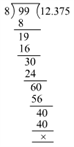We have:

$12 \frac {3} {8}$ = 12 + 0.375 = 12.375

(27) $2 \frac {19} {40}$

$2 \frac {19} {40}$ = $\frac {99} {40}$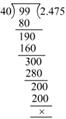We have:

$2 \frac {19} {40}$ = 2 + 0.475 = 2.475

(28) $\frac {19} {20}$

$\frac {19} {20}$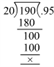We have:

$\frac {19} {20}$ = 0.95

(29) $\frac {37} {50}$

$\frac {37} {50}$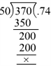We have:

$\frac {37} {50}$ = 0.74

(30) $\frac {107} {250}$

$\frac {107} {250}$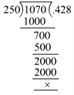We have:

$\frac {107} {250}$ = 0.428

(31) $\frac {3} {40}$

$\frac {3} {40}$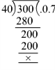We have:

$\frac {3} {40}$ = 0.075

(32) $\frac {7} {8}$

$\frac {7} {8}$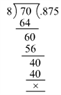We have:

$\frac {7} {8}$ = 0.875

(33) Using decimals, express

(i) 8 kg 640 g in kilograms

8 kg + 640 gm = 8 kg + $\frac {640} {1000} kg$

8 kg + 0.640 kg = 8.640 kg

(ii) 9 kg 37 g in kilograms

9 kg + 37 gm = 9 kg + $\frac {37} {1000} kg$

9 kg + 0.037 kg = 9.037 kg

(iii) 6 kg 8 g in kilograms

6 kg + 8 gm = 6kg + $\frac {8} {1000} kg$

6 kg + 0.008 kg = 6.008 kg

(34) Using decimals, express

(i) 4 km 365 m in in kilometers

4 km 365 m = 4 km + $\frac {365} {1000} \; km \; \left [ Since\; 1\; km\; =\; 1000\; m \right ]$

4 km + 0.365 km = 4.365 km

(ii) 5 km 87 m in kilometers

5 km 87 m = 5 km + $\frac {87} {1000} \; km \; \left [ Since\; 1\; km\; =\; 1000\; m \right ]$

5 km + 0.087 km = 5.087 km

(iii) 3 km 6 m in kilometers

3 km 6 m = 3 km + $\frac {6} {1000} \; km \; \left [ Since\; 1\; km\; =\; 1000\; m \right ]$

3 km + 0.006 km = 3.006 km

(iv) 270 m in kilometers

$\frac {270} {1000} \; km \; \; = 0.270\; km \; \left [ Since\; 1\; km\; =\; 1000\; m \right ]$

(v) 35 m in kilometers

$\frac {35} {1000} \; km \; = 0.035\; km \; \left [ Since\; 1\; km\; =\; 1000\; m \right ]$

(vi) 6 m in kilometers

$\frac {6} {1000} \; km \; = 0.006\; km \; \left [ Since\; 1\; km\; =\; 1000\; m \right ]$

(35) Using decimals, express

(i) 15 kg 850 g in kilograms

15 kg + 850 gm = 15 kg + $\frac {850} {1000} \; kg \; \left [ Since\; 1\; kg\; =\; 1000\; gm \right ]$

15 kg + 0.850 kg = 15.850 kg

(ii) 8 kg 96 g in kilograms

8 kg + 96 gm = 8 kg + $\frac {96} {1000} \; kg \; \left [ Since\; 1\; kg\; =\; 1000\; gm \right ]$

8 kg + 0.096 kg = 8.096 kg

(iii) 540 g in kilograms

540 gm = $\frac {540} {1000} = 0.540 \; kg \; \left [ Since\; 1\; kg\; =\; 1000\; gm \right ]$

(iv) 8 g in kilograms

8 gm = $\frac {8} {1000} = 0.008 \; kg \; \left [ Since\; 1\; kg\; =\; 1000\; gm \right ]$

(36) Using decimals, express:

(i) Rs 18 and 25 paise in rupees

Rs 18 + 25 paise = Rs 18 + $Rs\; \frac {25} {100} \; \left [ Since\;Re\; 1\;\; =\; 100\; paise \right ]$

Rs 18 + Rs 0.25 = Rs 18.25

(ii) Rs 9 and 8 paise in rupees

Rs 9 and 8 paise = Rs 9 + $Rs\; \frac {8} {100} \; \left [ Since\;Re\; 1\;\; =\; 100\; paise \right ]$

Rs 9 + Rs 0.08 = Rs 9.08

(iii) 32 paise in rupees

32 paise = Rs $Rs\; \frac {32} {100} = Rs\; 0.32 \; \left [ Since\;Re\; 1\;\; =\; 100\; paise \right ]$

(iv) 5 paise in rupees

5 paise = $Rs\; \frac {5} {100} = Rs\; 0.05 \; \left [ Since\;Re\; 1\;\; =\; 100\; paise \right ]$

Exercise 7C

(1) 9.6, 14.8, 37 and 5.9

9.6, 14.8, 37 and 5.9

Converting the decimals into like decimals:

9.6, 14.8, 37.0 and 5.9

Let us write the given numbers in the column form: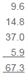Hence, the sum of the given numbers is 67.3

(2) 23.7, 106.94, 68.9 and 29.5

23.7, 106.94, 68.9 and 29.5

Converting the decimals into like decimals:

23.70, 106.94, 68.90 and 29.50

Let us write the given numbers in the column form: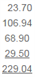Hence, the sum of the given numbers is 229.04

(3) 72.8, 7.68, 16.23 and 0.7

72.8, 7.68, 16.23 and 0.7

Converting the decimals into like decimals:

72.80, 7.68, 16.23 and 0.70

Let us write the given numbers in the column form:Hence, the sum of the given numbers is 97.41.

(4) 18.6, 84.75, 8.345 and 9.7

18.6, 84.75, 8.345 and 9.7

Converting the decimals into like decimals:

18.600, 84.750, 8.345 and 9.700

Let us write the given numbers in the column form: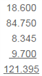Hence, the sum of the given numbers is 121.395

(5) 8.236, 16.064, 63.8 and 27.53

8.236, 16.064, 63.8 and 27.53

Converting the decimals into like decimals:

8.236, 16.064, 63.800 and 27.530

Let us write the given numbers in the column form: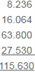Hence, the sum of the given numbers is 115.630.

(6) 28.9, 19.64, 123.697 and 0.354

28.9, 19.64, 123.697 and 0.354

Converting the decimals into like decimals:

28.900, 19.640, 123.697 and 0.354

Let us write the given numbers in the column form: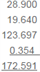Hence, the sum of the given numbers is 172.591

(7) 4.37, 9.638, 17.007 and 6.8

4.37, 9.638, 17.007 and 6.8

Converting the decimals into like decimals:

4.370, 9.638, 17.007 and 6.800

Let us write the given numbers in the column form: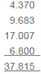Hence, the sum of the given numbers is 37.815.

(8) 14.5, 0.038, 118.573 and 6.84

14.5, 0.038, 118.573 and 6.84

Converting the decimals into like decimals:

14.500, 0.038, 118.573 and 6.840

Let us write the given numbers in the column form: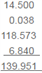Hence, the sum of the given numbers is 139.951

9. During three days of week, a rickshaw puller earns Rs 32.60, Rs 56.80 and Rs 72 respectively. What is his total earning during these days?

Earning on the 1st day of the week = Rs 32.60

Earning on the 2nd day of the week = Rs 56.80

Earning on the 3rd day of the week = Rs 72.00

Total earning = Rs 161.40

10. A man purchases an almirah for Rs 11025, gives Rs 172.50 as its cartage and spends Rs 64.80 on its repair. How much does the almirah cost him?

Cost of the almirah = Rs 11025.00

Money spend on cartage = Rs 172.50

Money spent on repair = Rs 64.800

Total cost of the almirah = Rs 11262.3

11. Ramesh covers 36 km 235 m by taxi, 4 km 85 m by rickshaw and 1 km 80 m on foot. What is the total distance covered by him?

Distance covered by the taxi = 36 km 235 m

Distance covered by the rickshaw = 4 km 085 m

Distance covered on foot = 1 km 080 m

Total distance covered = 41km 400m

12. A bag contains 45 kg 80 g of sugar and the mass of the empty bag is 950 g. What is the mass of the bag containing this much of sugar?

Weight of sugar in the bag = 45 kg 080 g

Weight of the empty bag = 0 kg 950 g

Total weight of the bag = 46kg 30g

13. Ramu bought 2 m 70 cm cloth for his shirt and 2 m 60 cm cloth of his pyjamas. Find the total length of cloth bought by him.

Length of the cloth for his shirt = 2 m 70 cm

Length of the cloth for his pyjamas = 2 m 60 cm

The total length of the cloth bought = 5m 30 cm

14. Radhika bought 2 m 5 cm cloth for her salwar and 3 m 35 cm cloth for her shirt. Find the total length of cloth bought by her.